# Fixed-point Iteration in MATLAB

• Fatima Hasan

#### Fatima Hasan

Homework Statement
The question is attached below.
Relevant Equations
-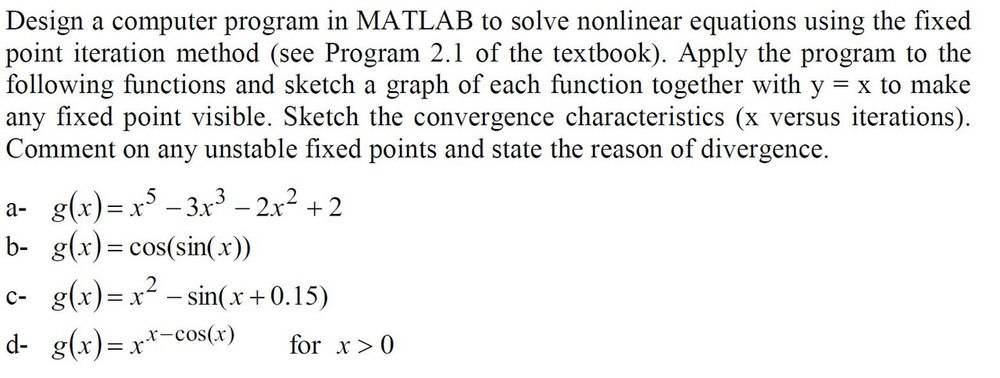I used the same code , but changing the equation ( line 4). In part (D) , I got a complex number , does it mean that the function is divergence ?
Here's my code :
Part c :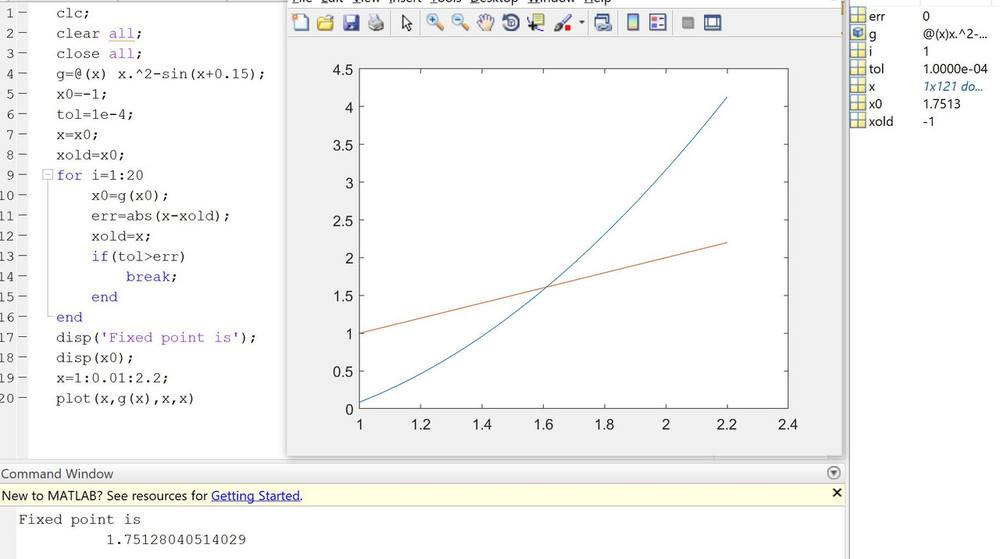Part d :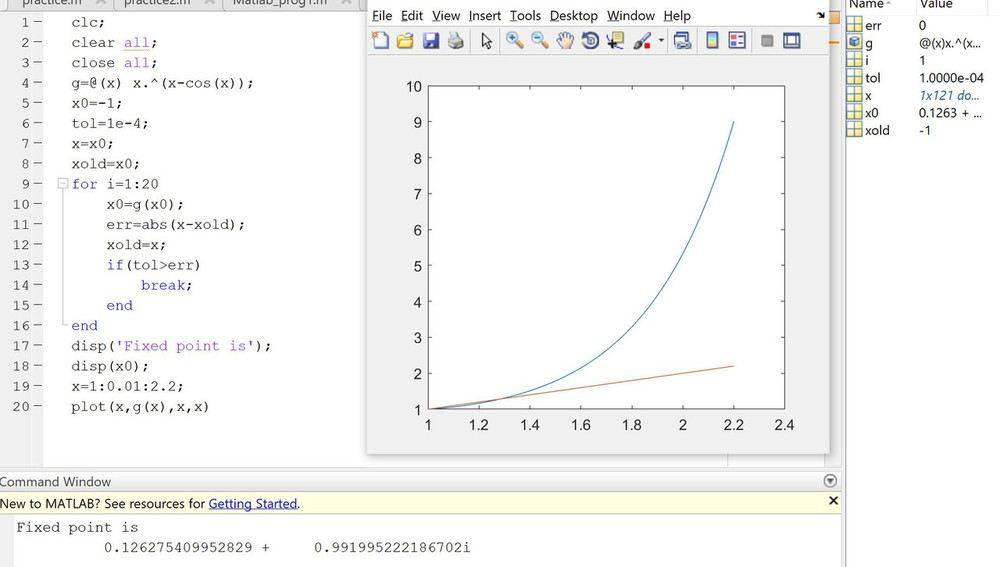Could someone confirm my answer please ? Any help would be greatly appreciated !

Matlab:
x0=g(x0)
How can your program work at all ? Or don't I understand Matlab ?
C)
Did you check with the plot ? where is g(x) = x ? Not at 1.75 !

D)
1^anything = 1 so how come that solution is not found ?

Matlab:
x0=g(x0)
How can your program work at all ? Or don't I understand Matlab ?
C)
Did you check with the plot ? where is g(x) = x ? Not at 1.75 !

D)
1^anything = 1 so how come that solution is not found ?
I set the initial conditions ( Lines : 4 - 8 ). The fixed point iteration in part C is x0=g(x0) and to compute the error ,we have to find the absolute value of the difference between x and xold. Once we have computed the error, the current value of x is stored in xold. x(1) is g(x0) when i =1 , x2 is g(x1) when i =2 , and so on ...

Does x0 = g(x0) change the value of x ?

Does x0 = g(x0) change the value of x ?
No, I think I have to write x instead of x0 in line 10 ,so x is varying in each iteration. Therefore , the error will be changed as well. Am I right now ?

Last edited:
Doesn't part d say "## \text{for} ~~ x > 0 ##"? I don't completely understand this, but is that right to start with ##-1##? Can you start with a different arbitrarily small number like ##1e-15##? I'm curious to see what happens and might even give it a go myself.

##x0 = g(x0)## looks right to me.

Last edited:
Doesn't part d say "## \text{for} ~~ x > 0 ##"? I don't completely understand this, but is that right to start with ##-1##? Can you start with a different arbitrarily small number like ##1e-15##? I'm curious to see what happens and might even give it a go myself.

##x0 = g(x0)## looks right to me.
I wrote the same code but changing the initial value of x0 , from ##-1## to ##1e-15## . Here's what I got :

Partc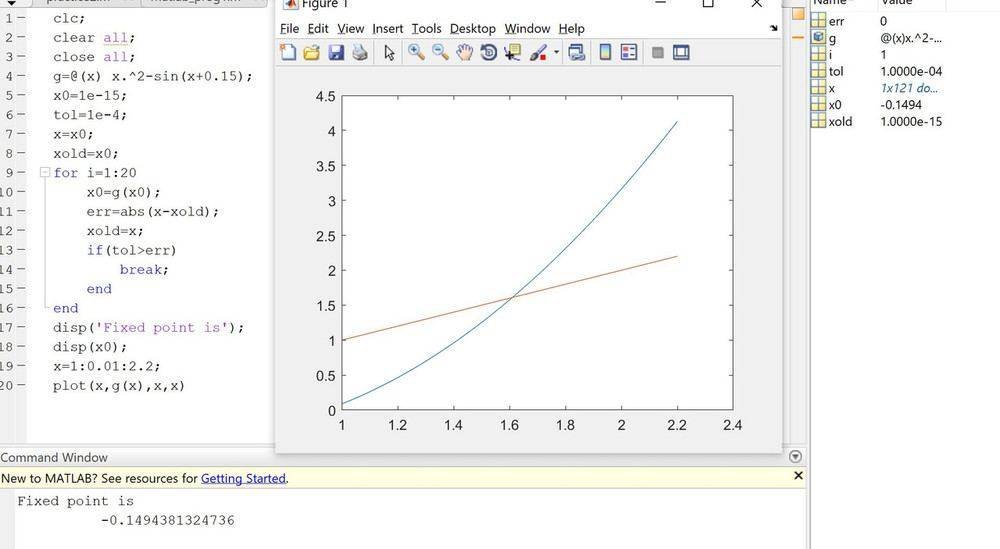Part d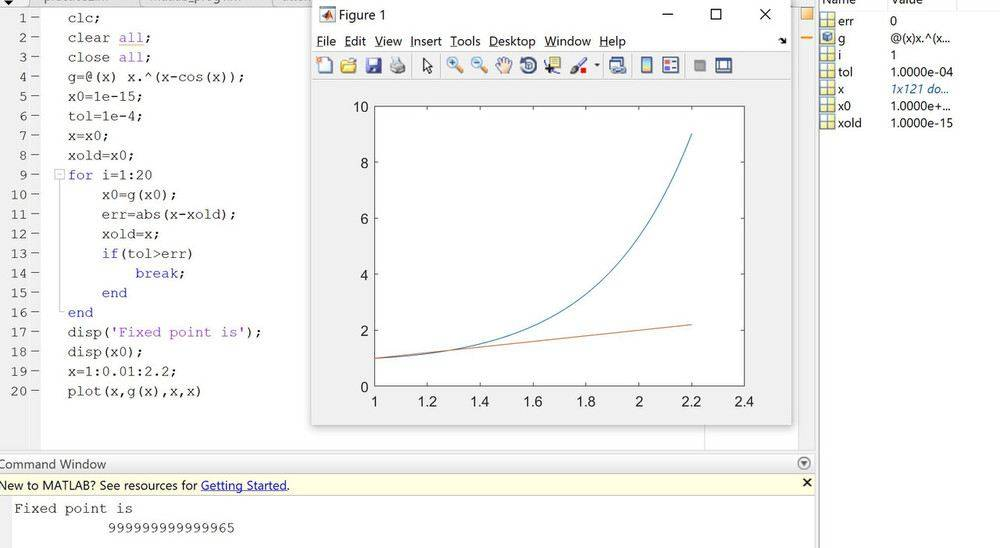Okay I see another thing (what others clearly noticed before me)... I was so concentrated on the function itself, but you do have another ##x## you're comparing ##x0## to when you check for your error; you're also plotting with respect to ##x##, which your code does not update. Make sure the ##x## and ##x0## is consistent.

A recommendation... I think a more elegant approach requires a modest change in your code. Change your ##x## into an array just like in line 19, but do this in the beginning at line 7 instead. Make the array something like:

Matlab:
x = x0.*ones(1, 20); % can be arbitrary numbers you're going to overwrite it anyways make sure your first one x(1) is x0

In the for loop you can refer to xold as ##x(i-1)## and the current iteration of x as ##x(i)## .

Matlab:
for i = 2:20 % start with 2 instead since your initialized version is x(1)

x(i) = g( x(i-1) ); % x(i) is your current x, and x(i-1) is xold
err = abs ( x(i) - x(i-1) );

if(tol > err)
break;
end
end

When you get to plotting... no need to overwrite x or to create a new array.

Okay I see another thing (what others clearly noticed before me)... I was so concentrated on the function itself, but you do have another ##x## you're comparing ##x0## to when you check for your error; you're also plotting with respect to ##x##, which your code does not update. Make sure the ##x## and ##x0## is consistent.

A recommendation... I think a more elegant approach requires a modest change in your code. Change your ##x## into an array just like in line 19, but do this in the beginning at line 7 instead. Make the array something like:

Matlab:
x = x0.*ones(1, 20); % can be arbitrary numbers you're going to overwrite it anyways make sure your first one x(1) is x0

In the for loop you can refer to xold as ##x(i-1)## and the current iteration of x as ##x(i)## .

Matlab:
for i = 2:20 % start with 2 instead since your initialized version is x(1)

x(i) = g( x(i-1) ); % x(i) is your current x, and x(i-1) is xold
err = abs ( x(i) - x(i-1) );

if(tol > err)
break;
end
end

When you get to plotting... no need to overwrite x or to create a new array.
Thanks for your reply. Could you please tell me how to write a MATLAB code in PF ?

I changed the code and that's what I got: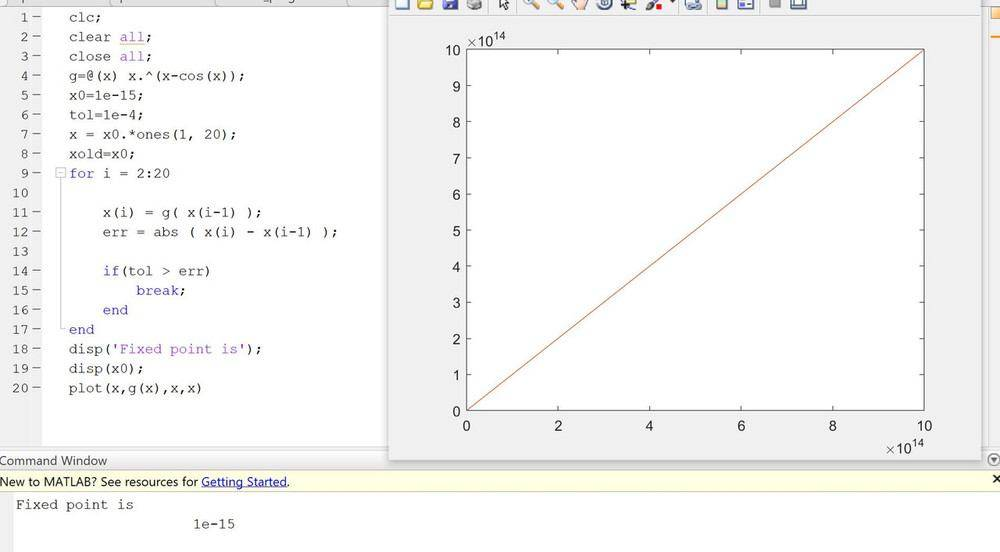I made a mistake changing ##x## into an array- my apologies (you can revert to your older version I think it worked better). I think your purpose for plotting both were to see where they intersect... shows that it should have converge.

There's an icon just above the text box.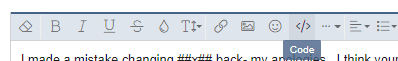I have no further feedback I think I'm going to have to fidget with this if I want to give you a better response. I might, but won't promise anything.

edit:

Did a little more digging. I think those last two are suppose to be difficult on purpose :) (difficult as in it won't converge easily) but it was fun to explore. That starting value really matters a lot for those two.

If I were to look at (roughly) ##x^2 - sin(x)## and I knew that ##x## was a really tiny magnitude ie. ##|x| << 1##... breaking it up into two parts I would know that ##x^2## is super tiny might be possible to neglect it and ##sin(x)## would be almost itself. If ##x## is large, then the sinusoidal is negligible and somehow ##x = x^2##. Good luck.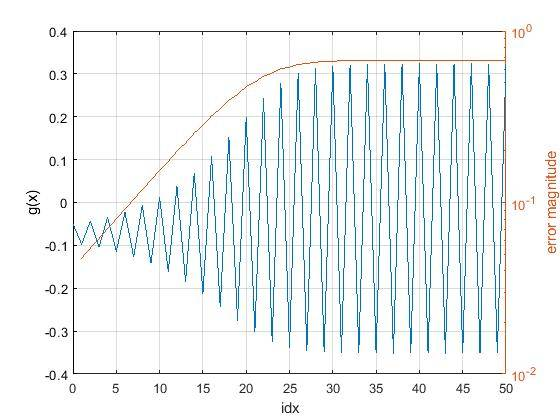Part d was really interesting. I should have looked at the formula... if you start with a tiny number like I suggested then it makes sense... ##x## is some small number to the power ##x-cos(x)## (probably a negative number), which makes it something like ##1 \over x^\text{small number}##. Of course it'll become ginormous just as we saw. Our trick for part c doesn't work here.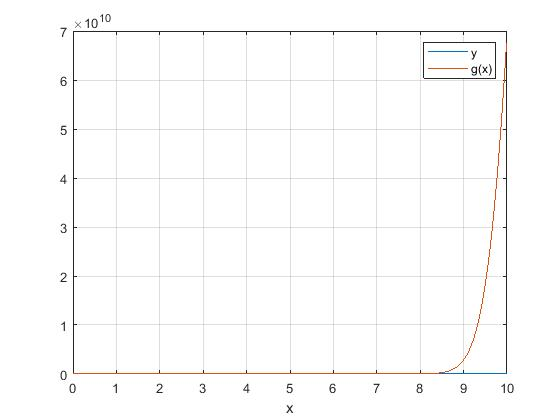(note the ##10^{10}## scale)

Your best bet is starting off with a bigger number, but how big? Funny enough... as you start close to 1 (+ or - minus a small fraction) then it's close to convergence. ##1^x##... it's going to be ##1##. You stray further away from that then ie ##0.5^{0.5-cos(0.5)}## and iterating it over and over again it's going to become some large number again.

Somewhere starting between 0.5 and 0.7 interesting things happen. I would recommend playing around there. I recommend plotting the error to see what's going on too ;) (this plot is when my starting value was 0.7).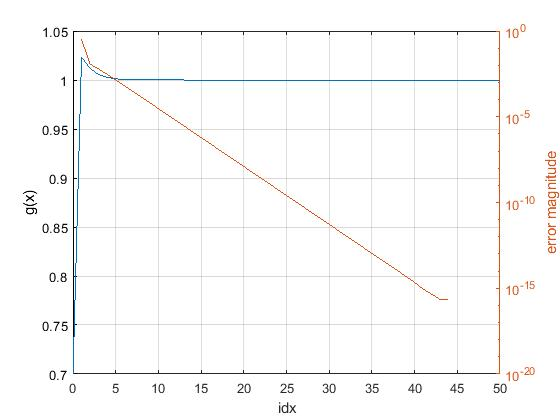Last edited: